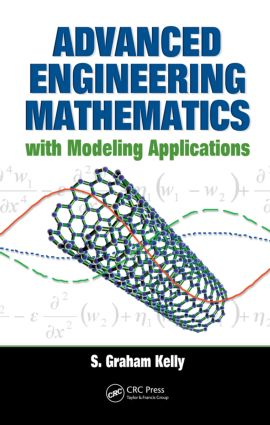# Advanced Engineering Mathematics with Modeling Applications

## 1st Edition

CRC Press

522 pages | 159 B/W Illus.

##### Purchasing Options:\$ = USD
Hardback: 9780849395338
pub: 2008-12-05
SAVE ~\$29.00
\$145.00
\$116.00
x
eBook (VitalSource) : 9780429126123
pub: 2008-12-05
from \$72.50

FREE Standard Shipping!

### Description

Engineers require a solid knowledge of the relationship between engineering applications and underlying mathematical theory. However, most books do not present sufficient theory, or they do not fully explain its importance and relevance in understanding those applications.

Advanced Engineering Mathematics with Modeling Applications employs a balanced approach to address this informational void, providing a solid comprehension of mathematical theory that will enhance understanding of applications – and vice versa. With a focus on modeling, this book illustrates why mathematical methods work, when they apply, and what their limitations are. Designed specifically for use in graduate-level courses, this book:

• Emphasizes mathematical modeling, dimensional analysis, scaling, and their application to macroscale and nanoscale problems
• Explores eigenvalue problems for discrete and continuous systems and many applications
• Develops and applies approximate methods, such as Rayleigh-Ritz and finite element methods
• Presents applications that use contemporary research in areas such as nanotechnology

Apply the Same Theory to Vastly Different Physical Problems

Presenting mathematical theory at an understandable level, this text explores topics from real and functional analysis, such as vector spaces, inner products, norms, and linear operators, to formulate mathematical models of engineering problems for both discrete and continuous systems. The author presents theorems and proofs, but without the full detail found in mathematical books, so that development of the theory does not obscure its application to engineering problems. He applies principles and theorems of linear algebra to derive solutions, including proofs of theorems when they are instructive. Tying mathematical theory to applications, this book provides engineering students with a strong foundation in mathematical terminology and methods.

### Table of Contents

Foundations of mathematical modeling

Engineering analysis

Conservation laws and mathematical modeling

Problem formulation

Nondimensionalization

Scaling

Linear algebra

Introduction

Three-dimensional space

Vector spaces

Linear independence

Basis and dimension

Inner products

Norms

Gram-Schmidt orthonormalization

Orthogonal expansions

Linear operators

Adjoint operators

Positive definite operators

Energy inner products

Ordinary differential equations

Linear differential equations

General theory for second-order differential equations

Differential equations with constant coefficients

Differential equations with variable coefficients

Singular points of second-order equations

Bessel functions

Differential equations whose solutions are expressible in terms of Bessel functions

Legendre functions

Variational methods

Introduction

The general variational problem

Variational solutions of operator equations

Finite-element method

Galerkin’s method

Eigenvalue problems

Eigenvalue and eigenvector problems

Eigenvalues of adjoint operators

Eigenvalues of positive definite operators

Eigenvalue problems for operators in finite-dimensional vector spaces

Second-order differential operators

Eigenvector expansions

Fourth-order differential operators

Differential operators with eigenvalues in boundary conditions

Eigenvalue problems involving Bessel functions

Eigenvalue problems in other infinite-dimensional vector spaces

Solvability conditions

Asymptotic approximations to solutions of eigenvalue problems

Rayleigh’s quotient

Rayleigh-Ritz method

Green’s functions

Partial differential equations

Homogeneous partial differential equations

Second-order steady-state problems, Laplace’s equation

Time-dependent problems: Initial value problems

Nonhomogeneous partial differential equations

Problems in cylindrical coordinates

Problems in spherical coordinates

Index

### Subject Categories

##### BISAC Subject Codes/Headings:
MAT003000
MATHEMATICS / Applied
TEC009000
TECHNOLOGY & ENGINEERING / Engineering (General)